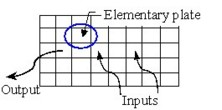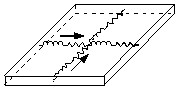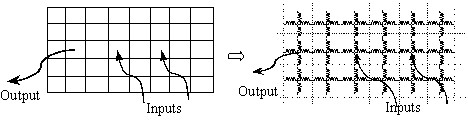# 2D mesh

## Introduction

The 2D mesh is used for plate conductors:

• sufficiently thin so that the variation of the current density on the thickness of the conductor can be neglected
• for which it is not easy to make an initial assumption on the path of the current flow

It is mainly the case of bus bars in power electronics where the input/output current terminals have small cross-section and the geometry of the studied device is flat.

## Principle

In the case of a 2D mesh, the planar conductor is split up into elementary plates of smaller sizes (see figure below).(An elementary plate is a 2D element of the mesh because the current has two directions of privileged flow: the two principal directions of the planar conductor).

An elementary plate is modeled by using four partial electric circuits (see figure below), in which a uniform current density is assumed.Using the previously described analytical formulas, it is possible to calculate: the partial resistance (Ri) and the partial inductance (Li) for each of four partial circuits, as well as partial mutual inductances (Mij) between the different elements.

Note: These values are independent from frequency.

## Equivalent electric scheme

The equivalent electric scheme of a plate solid conductor is obtained by associating the partial electric circuits of the elementary plates.

Note that there is a series connection between two resistors and two mutually coupled inductors at the interface of two meshes. To simplify the computation, the PEEC method automatically computes the values of the two resistors and two inductors and replaces them by equivalent single resistor and single inductor accordingly.The values of parameters of the plate solid conductor equivalent electric scheme depend on frequency.

## Note

By generating a mesh for each plate of the solid conductors, the 2D mesh can lead to a high number of elements, if this mesh is regular. Nevertheless, it is possible to apply another strategy of mesh making assumptions on the paths of the current lines, which is sometimes difficult to justify.